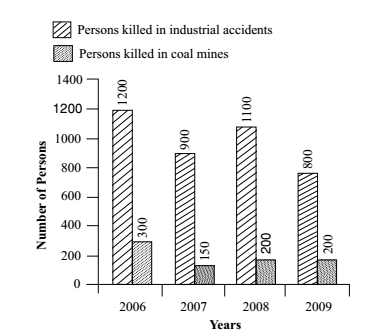Home » Data Interpretation » Bar graph » Question

#### Data Interpretation

Direction: Study the bar diagram and answer questions based on it.1. In a year, on an average, how many persons were killed in industrial accidents and coal mines together?
1. 121.25
2. 1212
3. 1212.5
4. 1000
##### Correct Option: C

Total number of persons killed in industrial accidents and coal mines in
2006 = 1200 + 300 = 1500
2007 = 900 + 150 = 1050
2008 = 1100 + 200 = 1300
2009 = 800 + 200 = 1000
Total number of persons killed
= 1500 + 1050 + 1300 + 1000 = 4850

 ∴ average = sum of the total number of persons killed in industrial accidents and coal mines total number of years

 = 4850 = 1212.5 4